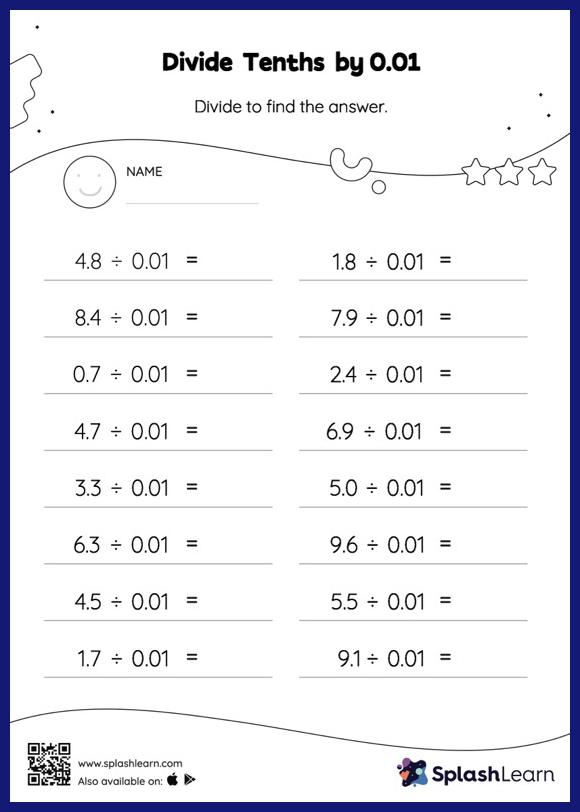# Divide Tenths by 0.01: Horizontal Division Worksheet

Home > Divide Tenths by 0.01: Horizontal DivisionLearners hone their math skills with this divide tenths by 0.01 Worksheet. When students divide two decimals, they do so without considering the decimal point at first. After that, they count the decimal digits in each number and add a decimal point at the appropriate place in the quotient. With the help of this divide tenths by 0.01 Worksheet, students can practice this idea in great depth.